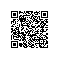# 大厂面试真题详解：稀疏矩阵乘法

Input:
[[1,0,0],[-1,0,3]]
[[7,0,0],[0,0,0],[0,0,1]]
Output:
[[7,0,0],[-7,0,3]]
Explanation:
A = [
[ 1, 0, 0],
[-1, 0, 3]
]

B = [
[ 7, 0, 0 ],
[ 0, 0, 0 ],
[ 0, 0, 1 ]
]

|  1 0 0 |   | 7 0 0 |   |  7 0 0 |
AB = | -1 0 3 | x | 0 0 0 | = | -7 0 3 |
| 0 0 1 |

Input:
[[1,0],[0,1]]
[[0,1],[1,0]]
Output:
[[0,1],[1,0]]

### 复杂度分析

public class Solution {
/**
* @param A: a sparse matrix
* @param B: a sparse matrix
* @return: the result of A * B
*/
public int[][] multiply(int[][] A, int[][] B) {
int rowA = A.length, columnA = A.length;
int rowB = B.length, columnB = B.length;
// AB为 rowA * columnB 大小的矩阵
int[][] AB = new int [rowA][columnB];
for (int i = 0; i < rowA; i++){
for (int j = 0; j < columnB; j++){
// 求出每一项
int sum = 0;
for (int k = 0; k < columnA; k++){
sum += A[i][k] * B[k][j];
}
AB[i][j] = sum;
}
}
return AB;
}
}使用钉钉扫一扫加入圈子
+ 订阅